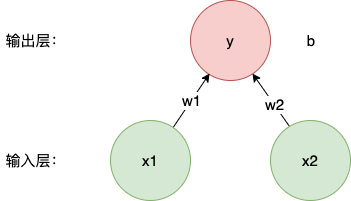# 使用 TensorFlow2.0 实现线性回归## 实现线性回归

1. 定义线性回归模型
2. 定义 Loss 函数
3. 定义迭代优化算法

### 定义线性回归模型

$\hat{y} = Xw + b$

import tensorflow as tf
import numpy as np
import random

def linear_reg(X, w, b):
# matmul 是矩阵乘法
return tf.matmul(X, w) + b


### 定义 Loss 函数

$Loss = \frac{1}{2n}(y-\hat{y})^2$

$Loss = \frac{1}{2n}(y-\hat{y})^{\top}(y-\hat{y})$
Loss 用 TF2.0 实现如下：

def squared_loss(y, y_hat, n):
y_observed = tf.reshape(y, y_hat.shape)
return tf.matmul(tf.transpose(y_observed - y_hat),
y_observed - y_hat) / 2 / n


### 定义迭代优化算法

SGD 算法每次会随机的从样本中选取一部分数据，例如每次取 100 条，然后计算这 100 条数据的 Loss，根据 Loss 求梯度，再用梯度来更新当前的参数，所以这里包含 3 个步骤：

1. 随机选择样本，每次选 n 条
2. 计算这 n 条样本的 Loss，并计算梯度，使用梯度更新参数
3. 循环 1 和 2

def data_iter(features, labels, mini_batch):
'''
数据迭代函数
Args:
- features: 特征矩阵 nxd 维
- labels: 样本，nx1 维
- mini_batch: 每次抽取的样本数
Example：
>>> mini_batch = 100
>>> for X, y in data_iter(features, labels, mini_batch):
'''
features = np.array(features)
labels = np.array(labels)
indeces = list(range(len(features)))
random.shuffle(indeces)
for i in range(0, len(indeces), mini_batch):
j = np.array(indeces[i:min(i+mini_batch, len(features))])
yield features[j], labels[j]


def sgd(params, lr):
'''
计算梯度，并更新模型参数
Args:
- params: 模型参数，本例中为 [w, b]
- lr: 学习率 learning rate
'''
for param in params:


# 产生模拟数据
# 1000 条样本，2 维特征
num_samples = 1000
num_dim = 2
# 真实的 weight, bias
w_real = [2, -3.4]
b_real = 4.2
# 产生特征，符合正态分布，标准差为 1
features = tf.random.normal((num_samples, num_dim), stddev=1)
labels = features[:,0]*w_real + features[:,1]*w_real + b_real
# 给 labels 加上噪声数据
labels += tf.random.normal(labels.shape, stddev=0.01)
# 学习率，迭代次数
lr = 0.03
num_epochs = 3
# 初始化模型参数
w = tf.Variable(tf.random.normal([num_dim, 1], stddev=0.01))
b = tf.Variable(tf.zeros(1,))
mini_batch = 10
# 开始训练
for i in range(num_epochs):
for X, y in data_iter(features, labels, mini_batch):
# 在内存中记录梯度过程
t.watch([w, b])
# 计算本次小批量的 loss
l = squared_loss(y, linear_reg(X, w, b), mini_batch)
# 计算梯度，更新参数
sgd([w, b], lr)
# 计算本次迭代的总误差
train_loss = squared_loss(labels, linear_reg(features, w, b), len(features))
print('epoch %d, loss %f' % (i + 1, tf.reduce_mean(train_loss)))


## 简单实现

from tensorflow import keras
from tensorflow.keras import layers
from tensorflow import initializers as init

model = keras.Sequential()


from tensorflow import losses

loss = losses.MeanSquaredError()


from tensorflow.keras import optimizers

trainer = optimizers.SGD(learning_rate=0.03)


from tensorflow import data as tfdata

batch_size = 10
dataset = tfdata.Dataset.from_tensor_slices((features, labels))
dataset = dataset.shuffle(len(features)).batch(batch_size)


from tensorflow import data as tfdata
from tensorflow import keras
from tensorflow.keras import layers
from tensorflow import initializers as init
from tensorflow import losses
from tensorflow.keras import optimizers

# 设置网络结构：1 层全连接，初始化模型参数
model = keras.Sequential()
# loss 函数：MSE
loss = losses.MeanSquaredError()
# 优化策略：随机梯度下降
trainer = optimizers.SGD(learning_rate=0.03)
# 设置数据集，和小批量的样本数
batch_size = 10
dataset = tfdata.Dataset.from_tensor_slices((features, labels))
dataset = dataset.shuffle(len(features)).batch(batch_size)

num_epochs = 3
for epoch in range(1, num_epochs+1):
# 取小批量进行计算
for (batch, (X, y)) in enumerate(dataset):
# 计算 loss
l = loss(model(X, training=True), y)
# 计算梯度并更新参数

# 本次迭代后的总 loss
l = loss(model(features), labels)
print('epoch %d, loss: %f' % (epoch, l.numpy().mean()))
# 输出模型参数
print(model.get_weights())


## 小结

1. 按照定义模型、定义损失函数，以及定义迭代算法这几个基本的步骤来实现一个广义的神经网络，麻雀虽小，但五脏俱全
2. 使用丰富的 TF2.0 组件来实现一个更精简的版本，旨在了解 TF2.0 的使用。©️2019 CSDN 皮肤主题: 数字20 设计师: CSDN官方博客